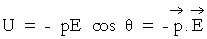# Short Notes: Electric Potential and Potential Energy# Definition of Electric Potential

For defining electric potential we take the reference of zero potential at infinity.

The electric potential at any point in an electric field is defined as the work done in bringing per unit (infinitesimal) positive test charge from infinity to given point. Therefore, if W is the work done is bringing test charge q0 from infinity to given point in an electric field, then electric potential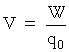q is taken as infinitesimal so that it may not affect the original electric field; hence it is better to writeDefinition of Electric Potential Difference : The electric potential difference between two points in an electric field is defined as the work done in bringing per unit infinitesimal positive test charge from one point to another against the direction of electric field.If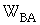is the work done in bringing the positive test charge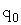from B to A, then electric potential difference between A and B is# Units and Dimensions of Electric Potential

The electric potentialThe S.I. unit of work ( W ) is joule and of charge ( q0 ) is coulomb, so S.I. unit of electric potential is joule/coulomb or volt.

Dimensional formula for work is  [ML2T-2]and of q0 is [AT], so dimensional formula for electric potential is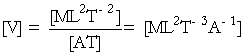# Relation between electric field and potential

Let dV = potential difference between A and B.

= work done in taking unit +ve charge from  A → B  or from B → A against the field

=  E. dr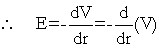Negative gradient of potential is electric field.

# Physical Meaning of Electric Potential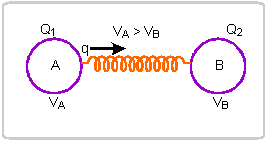The electric potential is that physical quantity which determines the direction of flow of charge from one body to another when brought in electrical contact.

If two charged bodies A and B have charges Q and QB and potentials VA and VA respectively such that VA > VA , then when bodies A and B are brought in electrical contact, the charge will always flow from body A to body till then potentials become equal; whatever the initial charges on bodies A and B may be.

# Electric Potential as Line Integral of Electric FieldThe electric potential at any point in an electric field is given by the negative line integral of electric field from infinity to given point in electric field.

If P is a point in electric field of a distance r andis electric field at intermediate length element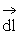(at point A ) betweenand point P, then electric potential at P isThis line integral is independent of path followed between infinity and point P.

# Electric Potential due to a point charge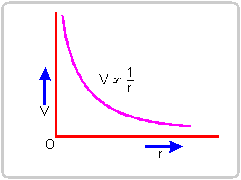The electric potential due to a point charge at a any point is defined as the work done in bringing per unit (infinitesimal and positive) test charge from infinity to given point. Let q be the charge and P a point at a distance r from q at which the potential is to be found.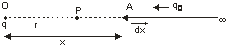Suppose test chargeis being brought from infinity to point P . When test chargeis at a distance x, the electrostatic coulomb force on the test charge is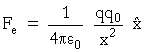whereis unit vector along.

Work done by external force against electric force during small displacement dx isWork done in bringing test chargefrom infinity to point P is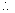The electric potential at point P (distant r from point charge q ) isClearly, the potential due to a point charge varies inversely with distance r from the point charge (fig.)

# Electric Potential due to a system of Charges

The electric potential at a point due to a system of point charges is equal to the algebraic sum of potentials due to all individual charges of the system at that point. Therefore, if  V1,V2,V3,……..,Vare the electric potentials at given point due to charges  q1,q2,q3,……. qn of the system, then the net electric potential at that point will beWhile calculating potential at a point it should be taken into account what type of charge is it. If it is positive charge, potential is positive. If it is negative charge, potential is negative.

# Electric potential due to an Electric Dipole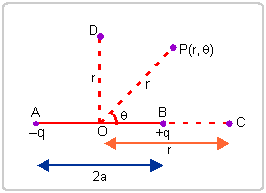Theis electric dipole moment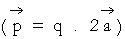of dipole formed of charge ? q and + q at separation 2 a , then the electric potential at a distance r from the centre of dipole at difference positions are given by

(i) At Axial point, C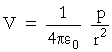(ii) At equatorial point D ,

V =0     (zero)

(iii) At any general point P having polar coordination  (r,θ)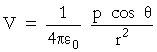.

# Equipotential Surface

The surface having same electric potential at its each point is called an equipotential surface. The surface of a charged conductor is always an equipotential surface.

Charactersitics :

(i) The electric field lines are always perpendicular to every point of equipotential surface.

(ii) The work done in moving a charge between any two points on equipotential surface is always zero.

# Electric Potential Energy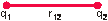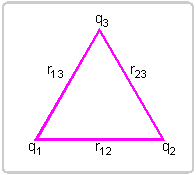The electric potential energy of a system of charges is defined as the work done in assembling the system of charges assuming them initially at infinity from one another.

(i) For a system of two charges : If two charges q and q are at a distance r12,  then electric potential energy is given by(ii) For a system of three charges :(iii) For a system of n -charges :# Electric Potential Energy of an Electric Dipole in a Uniform Electric FieldThe electric potential energy of an electric dipole in an electric field is defined as the work done by external force in bringing the dipole from infinity to its present position and orientation. If p is dipole moment and E is the electric field and θ is angle between the directions ofand, then the electric potential energy of dipole